# Yang-Baxter equation

Letbe an-matrix with entries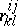. Viewas an endomorphism of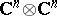with basis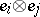(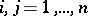), so that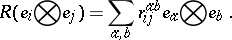(a1)

The constant Yang–Baxter equation, or quantum Yang–Baxter equation, foris: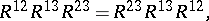(a2)

where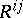is the endomorphism of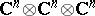acting likeon the two factorsand leaving the third factor alone. Thus,,and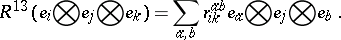(a3)

An alternative form of the Yang–Baxter equation is: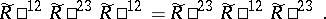(a4)is a solution of (a2) if and only ifis a solution of (a4), whereis the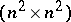-matrix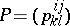,, which switches the two factors of.

The Yang–Baxter equation (a2) can be interpreted as a condition on the interaction of relativistic particles with internal state space, [a2]. Let the interaction between two particles with rapidities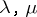be given by; then the Yang–Baxter equation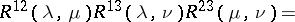(a5)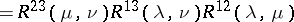expresses the condition that the interaction between three particles is determined by the two particle interactions and is independent of which particles interact first. In this connection the Yang–Baxter equation is also called the factorization equation. It occurred first in [a5], [a6]. In lattice statistical mechanics there is another interpretation of the Yang–Baxter equation, [a3], [a4]. In this context it is also called the triangle equation or the star-triangle equation. Its sources go back to [a7].

If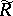satisfies (a4), let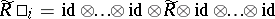, where there are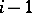factors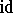beforeand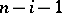factorsafter. Then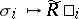defines a representation of the braid group, whereis the-th simple braid, cf. Braid theory. This is one main reason for the interconnection between knot and braid theory on the one hand and quantum field theory and statistical mechanics on the other, cf. e.g. [a9].

A generalization of the Yang–Baxter equation are the fundamental commutation relations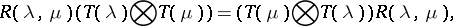(a6)

introduced by L.D. Faddeev, E.K. Sklyanin, L.A. Takhtazhyan as a basis for their quantum inverse scattering method, [a8]. In (a6),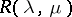is an-matrix of scalars (as before; usually satisfying the Yang–Baxter equation) andis an-matrix of operators. Equation (a6) serves as a starting point for using an (algebraic) Bethe Ansatz for calculating eigenvalues and eigenvectors of the Hamiltonian operator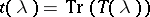. Solutions of (a6) relate to families of representations of certain Hopf algebras, in particular (duals of) quasi-triangular Hopf algebras, cf. Quantum groups and [a14], [a15], [a11].

The classical Yang–Baxter equation is the semi-classical limit of (a2). It is(a7)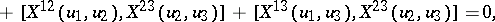whereis a function with values in, whereis a Lie algebra. (To interpret (a7), imbedin its universal enveloping algebra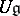: the meaning of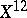, etc. is analogous to the case of equation (a2).) The classical Yang–Baxter equation has much to do with completely-integrable Hamiltonian systems (cf. Hamiltonian system; Soliton), cf. [a12], and the results of [a13] go some way to explaining why solutions to soliton equations typically involve principally elliptic, trigonometric and rational functions.

How to Cite This Entry:
Yang-Baxter equation. Encyclopedia of Mathematics. URL: http://encyclopediaofmath.org/index.php?title=Yang-Baxter_equation&oldid=23155